Courses

# Metal Oxide Semiconductor Field Effect Transistor (MOSFET) I-V Characteristics Electrical Engineering (EE) Notes | EduRev

## VLSI System Design

Created by: Machine Experts

## Electrical Engineering (EE) : Metal Oxide Semiconductor Field Effect Transistor (MOSFET) I-V Characteristics Electrical Engineering (EE) Notes | EduRev

The document Metal Oxide Semiconductor Field Effect Transistor (MOSFET) I-V Characteristics Electrical Engineering (EE) Notes | EduRev is a part of the Electrical Engineering (EE) Course VLSI System Design.
All you need of Electrical Engineering (EE) at this link: Electrical Engineering (EE)

Objectives

In this course you will learn the following

• Derivation of I-V relationship
• Channel length modulation and body bias effect

6.1 derivation of I-V relationship

In this section, the relation between IDS and VGS is discussed. We assume that gate-body voltage drop is more than threshold voltage Vr, so that mobile electrons are created in the channel. This implies that the transistor is either in linear or saturationregion.

Here we will derive some simple I-V characteristics of MOSFET, assuming that the device essentially acts as a variable resistor between source and drain, and only drift ohmic current needs to be calculated. Also note that the MOSFET is basically a two-dimensional device. The gate voltageVGS produces a field in the vertical (x) direction, which induces charge in the silicon, including charge in the inversion layer. The voltage VDS produces a field in the lateral (y) direction, and current flows (predominantly) in the y-direction. Strictly speaking, we must solve the 2-D Poisson and continuity equations to evaluate the I-Vcharacteristics of the device. These are analytically intractable. We therefore resort to the gradual channel approximation described below.

To find the current flowing in the MOS transistor, we need to know the charge in the inversion layer. This charge, Qn(y) (per sq. cm ) is a function of position along the channel, since the potential varies going from source to drain. We assume that Qn(y) can be found at any point y by solving the Poisson equation only in the x direction, that is treating the gate-oxide-silcon system in the channel region very much like a MOS capacitor. This is equivalent to assuming that vertical electric field Ex is much larger than the horizontal electric field Ey, so that the solution of the 1-dimensional Poisson equation is adequate. This gradual channel approximation (the voltage varies only gradually along the channel) is quite valid for long channel MOSFETs since Ey is small. For Qn(y) using charge control relation at location we have :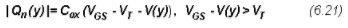Now we turn our attention to evaluate the resistance of the infinitesimal element of length dalong the channel(as shown in fig 6.21). Assuming that only drift current is present and hence applying Ohm's law, we get :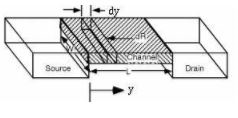Fig 6.21: Cross Sectional View of channel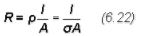Here we have l = dy,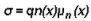and A=Wxi, where xi=inversion layer thickness.

Now using equation (6.22), We have :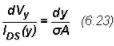Since σ is varying along the transverse direction, we define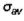as :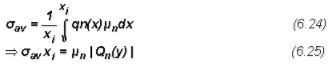Now usingin eqn (6.23) and rearranging the terms,we will get :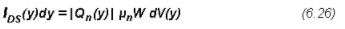Neglecting recombination-regeneration which implies IDS(y) = IDS i.e. current constant throughtout the channel.

Integrating RHS of eqn (6.26) from 0 to VDS and LHS from 0 to L, we will get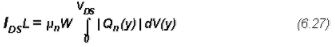Now substituting Qn(y) from eqn (6.21) in eqn (6.27), we will get :Eqn (6.29) holds true for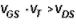.

The drain current first increases linearly with the applied drain-to-source voltage, but then reaches a maximum value. This occurs due to the formation of depletion region between pinch-off point and drain. This behaviour is known as drain saturation which is observed for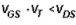as shown in figure below.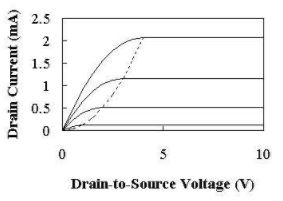Fig 6.22: IDS-VDS graph

The saturation current IDSsat is given by eqn (6.210),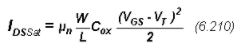6.2 Channel length modulation and body bias effect

The observed current IDS does not saturate, but has a small finite slope as shown in fig 6.31. This is attributed as channel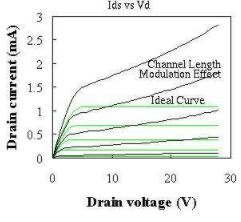Fig 6.31: Actual vs Ideal IDS-VDS graph

length modulation. This in MOSFET is caused by the increase in depletion layer width at the drain as the drain voltage is increased. This leads to a shorter channel length (reduced by ΔL) and increased drain current. When the channel length of MOSFET is decreased and MOSFET is operated beyond channel pinch-off, the relative importance of pinch-off length ΔL with respect to physical length is increased. This effect can be included in saturation current as :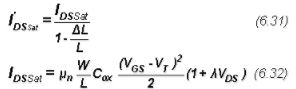Here λ is called channel length modulation coefficient.

Till now we assumed that the body of MOSFET is to be grounded. We will now take effect of body bias into account i.e. body being applied a negative voltage in case of n-MOSFET. Application of VSB > 0 increases the potential build up across the semiconductor. Depletion region widens in order to compensate for the extra required field, which implies higher VT. Viewing it from the point of energy band diagram, a higher potential needs to be applied to the gate in order to bend the bands by the same ammount in order to create the same electron concentration in the channel. With the application to the body bias, it modulates to the threshold voltage governed by the threshold voltage governed by the following equations :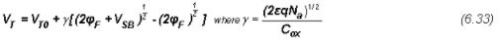where γ is known as the body coefficient.

Offer running on EduRev: Apply code STAYHOME200 to get INR 200 off on our premium plan EduRev Infinity!

,

,

,

,

,

,

,

,

,

,

,

,

,

,

,

,

,

,

,

,

,

;